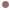Autres Articles de :
3D flows

# 2007 A 3D symmetrical toroidal chaos

Christophe LETELLIER
17/04/2009
Dequan Li

There are various types of chaotic dynamics. In three dimensions they have been distinguished by their global topologies, that is, the structure of the phase space that contains their chaotic attractors. Among all known chaotic attractors produced by autonomous systems, there are very few toroidal chaotic attractors , but none exhibits a symmetry. A set of ordinary differential equations proposed by Li produces a new chaotic attractor with a rotation symmetry and a nontrivial toroidal structure.The system

The set of three ordinary differential equations recently proposed by Dequan Li  is :This system of equations is invariant under the group of two-fold rotations about the symmetry axis in the phase space R3 (x,y,z) :. It was modeled after the Lorenz system, but contains two additional symmetry-preserving terms : dxz in the first equation and -ex2 in the third equation.

This system has three fixed points, one located on the symmetry axis at the origin (0,0,0), and two symmetry-related fixed points. If we defineandbythe symmetric fixed points are.Fig. 1 : A non trivial quasi-periodic regime

 Bo Deng, Constructing homoclinic orbits and chaotic attractors, International Journal of Bifurcation & Chaos, 4, 823-841, 1994.

 Dequan Li, A three-scroll chaotic attractor, Physics Letters A, 372, 387-393, 2007.